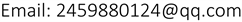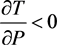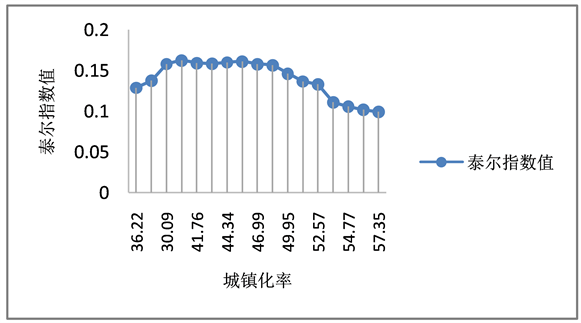﻿ 城镇化与城乡收入差距的倒“U”关系的研究 Research on the Inverted “U” Relationship between Urbanization and Rural-Urban Income Gap

Vol.07 No.07(2018), Article ID:26100,8 pages
10.12677/ASS.2018.77160

Research on the Inverted “U” Relationship between Urbanization and Rural-Urban Income Gap

Yu Liu

Jiangxi University of Finance and Economics, Nanchang JiangxiReceived: Jul. 5th, 2018; accepted: Jul. 19th, 2018; published: Jul. 26th, 2018ABSTRACT

In the past forty years of reform and opening up, China’s economic development has been greatly improved, the per capita disposable income has been greatly improved, and the per capita income has entered the ranks of the middle-income countries. At the same time, the absolute poverty population is decreasing and the level of urbanization is improving. However, the people do not enjoy equal development results with the rapid economic growth in China. The gap between the rich and the poor in China is still in a higher position. In recent years, the income gap between urban and rural areas in China has shown a great fluctuation, resulting in a series of social problems. Conflicts of interest among all strata are constantly showing up, which is not conducive to the construction of a harmonious society. This paper uses mathematical deduction and national panel data to analyze the impact of urbanization level on urban-rural gap in China at the present stage, which is based on the Theil index model. Using national time series data, the paper empirically tests the relationship between urbanization level and urban and rural income gap and its internal coordination mechanism; and aiming at the current situation of income gap urban and rural areas in China, it also puts forward the problems that should be paid attention to in the process of urbanization, and how to narrow the gap between urban and rural income and avoid the negative impact of urban and rural differentiation.

Keywords:Urban-Rural Income Gap, Urbanization Level, Theil Index1. 文献回顾

1.1. 国外研究现状

1.2. 国内研究现状

2. 城镇化率与城乡收入差距倒U关系的数理推导

2.1. 变量解释

2.1.1. 被解释变量：城乡收入差距

2.1.2. 解释变量：城镇化率

2.2. 数理证明的推导过程

${I}_{1}=\frac{y{N}_{1}}{y{N}_{1}+x{N}_{2}}=\frac{yP}{yP+x\left(1-P\right)}$，农村人口收入比为$T={I}_{1}×\mathrm{ln}\frac{{I}_{1}}{{P}_{2}}+{I}_{2}×\mathrm{ln}\frac{{I}_{2}}{{P}_{2}}={I}_{1}×\mathrm{ln}\frac{{I}_{1}}{P}+\left(1-{I}_{1}\right)×\mathrm{ln}\left(\frac{1-{I}_{1}}{1-P}\right)$ (1)

${I}_{1}$ 代入(1)式得

$T=\frac{yp}{yp+x\left(1-p\right)}\text{ln}\frac{y}{x}+\text{ln}\frac{x}{yp+x\left(1-p\right)}$ : (2)

a：对(2)式中x求偏导得：

$\frac{\partial T}{\partial x}=-\frac{yP\left(\mathrm{ln}y-\mathrm{ln}x\right)\left(1-P\right)}{{\left[yp+x\left(1-P\right)\right]}^{2}}$ , (3)

$\frac{\partial T}{\partial y}=\frac{xP\left(1-P\right)\left(\mathrm{ln}y-\mathrm{ln}x\right)}{{\left(yP+x-xP\right)}^{2}}$ ; (4)

b：求(2)式中关于P的一阶偏导，此时将 $x,y$ 看成常数，

 时，T达到最大值，此时 ${P}_{\text{e}}=\frac{xy\mathrm{ln}\frac{y}{x}-xy+{x}^{2}}{{\left(y-x\right)}^{2}}$

$P<{P}_{e}$ 时， $\frac{\partial T}{\partial P}>0$，则T关于P单调递增；泰尔指数随着城镇化率的增加而增加；

$P>{P}_{e}$ 时，，则T关于P单调递减，泰尔指数随着城镇化率的增加而减少。

${P}_{e}$ 是城乡收入差距先增大后缩小的拐点。

2.3. 结论与原因分析

2.3.1. 结论

2.3.2. 原因分析

3. 城镇化率与城乡收入差距倒“U”关系的实例分析

$T=\frac{yP}{yP+x\left(1-P\right)}\mathrm{ln}\frac{y}{x}+\mathrm{ln}\frac{x}{yP+x\left(1-P\right)}$，根据表1数据计算城乡人口数以及城镇化率，计算得出泰尔指数Table 1. Urban and rural residents’ income, urbanization rate and Theil index in 2000~2016 yearsFigure 1. National Theil index trend map

4. 缩小城乡收入差距的措施

4.1. 进一步深化城乡户籍制度改革

4.2. 积极推进新型城镇化，用科学发展观来统筹城镇化建设

4.3. 降低公共服务的差异化，扩大社会保障与社会福利的覆盖范围

Research on the Inverted “U” Relationship between Urbanization and Rural-Urban Income Gap[J]. 社会科学前沿, 2018, 07(07): 1072-1079. https://doi.org/10.12677/ASS.2018.77160

1. 1. Ledrman, D. and Bravo-ortega, C. (2004) Is There an Urban Bias in Public Expenditure: A Welfare Approach. Columbia University Press, New York.

2. 2. Hertel, T. and Fan, Z. (2006) Labor Market Distortions, Rural-Urban Inequality and the Opening of China’s Economy. Economic Modelling, 23, 76-109. https://doi.org/10.1016/j.econmod.2005.08.004

3. 3. Wang, Z.X., Smyth, R. and Huang, Y.G. (2009) A New Ordered Family of Lorenz Curves with an Application to Measuring Income Inequality and Poverty in Rural China. China Economical Review, 20, 218-235. https://doi.org/10.1016/j.chieco.2008.12.003

4. 4. Treiaman, D.J. (2012) The Difference between Heaven and Earth: Ur-ban-Rural Disparities in Well-Being in China. Research in Social Stratification and Mobility, 30, 33-47. https://doi.org/10.1016/j.rssm.2011.10.001

5. 5. 杨森平. 我国城乡收入差距与城镇化率的倒U关系研究[J]. 经济与金融管理, 2015, 27(11): 44-48.

6. 6. 洪丽, 尹康. 中国城镇化与城乡收入差距的“倒U”型拐点测度——基于东、中、西部地区省际面板数据的实证研究[J]. 统计与信息论坛, 2015, 30(9): 12-21.

7. 7. 穆怀中, 吴鹏. 城镇化、产业结构优化与城乡收入差距[J]. 经济学家, 2016(5): 37-44.

8. 8. 吕炜, 高飞. 城镇化、市民化与城乡收入差距——双重二元结构下市民化措施的比较与选择[J]. 财贸经济, 2013, 34(12): 38-46.

9. 9. 傅振邦, 陈先勇. 城市化、产业结构变化与城乡收入差距——以湖北省为例[J]. 中南财经政法大学学报, 2012(6): 8-14.

10. 10. 李权葆, 薛欣. 城乡基尼系数测算与收入分配差距分析——基于CHNS的实证分析[J]. 管理评价, 2013, 25(3): 82-89.